Дана: 0

Барлыгы: 0,00

0

# Surface area of spheres (demonstration)

### Surface area of spheres (demonstration)

The surface of a sphere consists of the set of points which are all at the same distance from a given point in space.

Математика

Этикеткалар

surface of sphere, great circle, математика, геометрия, surface

Байланысты экстралар

### Көріністер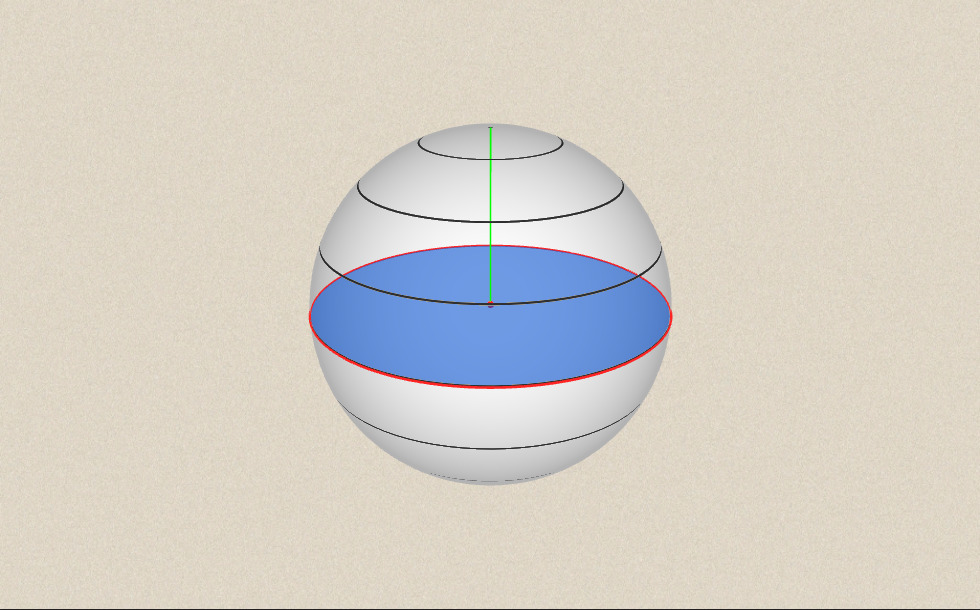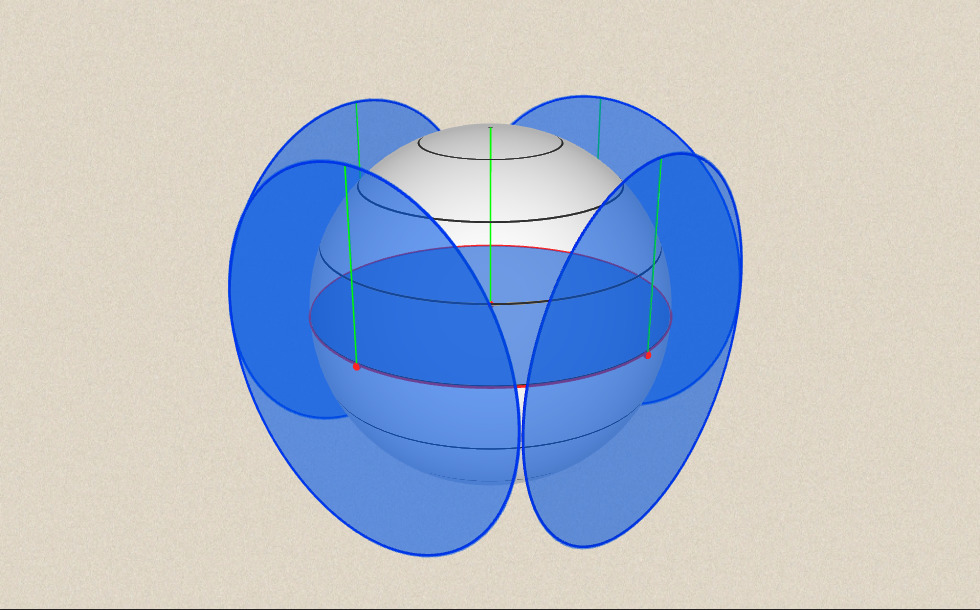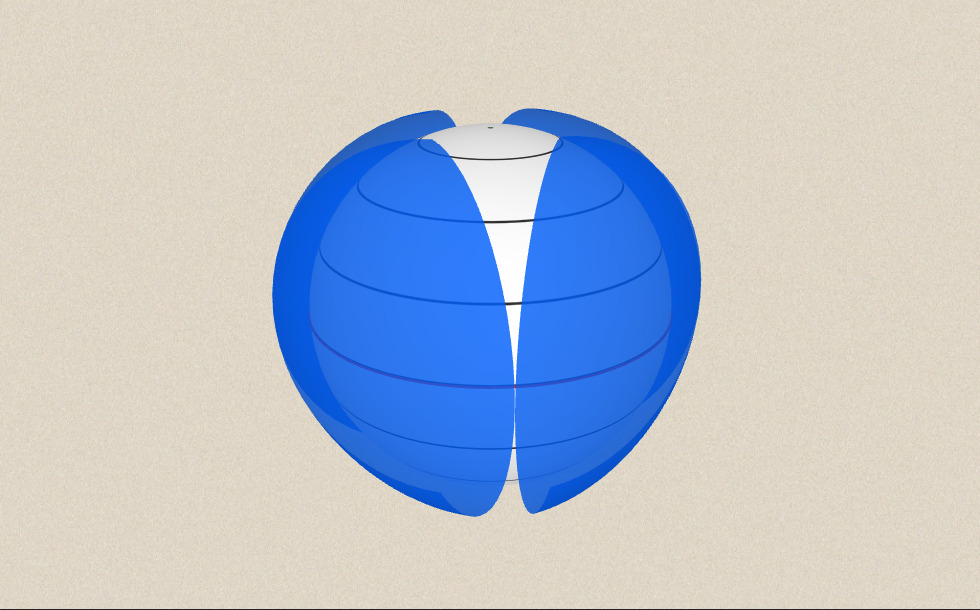### Байланысты экстралар#### Sphere

A sphere is the set of points which are all within the same distance from a given point...#### Жазық фигуралардың периметрі мен ауданын, беттің ауданын және көлемін есептеу

Анимацияның көмегімен жазық фигуралардың периметрі мен ауданын есептеу формуласымен,...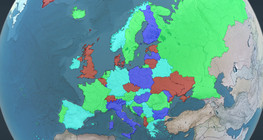#### Four colour theorem

Colour a map with the fewest number of colours possible, so that no two adjacent regions...#### Non-orientable surfaces

The Möbius strip and the Klein bottle are special two-dimensional surfaces with only one...#### Volume of spheres (Cavalieri´s principle)

Calculating the volume of a sphere is possible using an appropriate cylinder and cone.#### Volume of spheres (demonstration)

The sum of the volume of the ´tetrahedrons´ gives an approximation of the volume of the...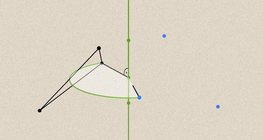#### Geometric transformations – rotation

This animation demonstrates geometric rotation, a type of geometric transformation both...#### Solids of revolution

Rotating a geometric shape around a line within its geometric plane as an axis results in...